# Understanding Markov Chains

#### …and their connections to Neuroscience, AI and Russian Poetry

“My dreams, my dreams! What has become of their sweetness? What indeed has become of my youth?”
― Alexander Pushkin, Eugene Onegin

It might seem strange to start an article on Markov chains (also called Markov processes) with a quote from a 19th-century Russian poem, but there is a surprising connection between the two of them that was at the beginning of the development of an entirely new field of probability theory at the beginning of the 20th century.

Today, Markov chains are everywhere. It is impossible to imagine AI without them, and applications range from natural language processing, voice recognition, web search, and protein discovery to self-driving cars.

But before we delve into the connection between Markov chains and Russian poetry, I want to start out more generally with some points on importance of stochastic processes, and there is no better place to begin than with the concept of a random walk.

As is frequently the case in science, ideas seem to be hanging around in the air and are often discovered by several thinkers independently in a span of years or even months. Something similar took place at the turn of the 19th century when scientists increasingly became aware of the importance of stochastic processes such as random walks. In a span of years, random walks popped up in the context of mosquito populations (where they were tied to the spread of disease), Brownian motion of molecules (part of Einstein’s annus mirabilis), acoustics, and the financial market.

To start formally, a random walk is a stochastic process that describes the path of a subject in a mathematical space, which can be constituted by something like the integers, a 2-dimensional or higher-dimensional Euclidian space, but also many other constructs. Starting from an initial state, we take n steps by adding up random variables drawn from a probability distribution at each step: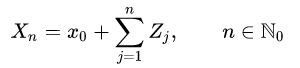X is the position in mathematical space, n is the number of steps, and Zj are random variables distributed following a certain distribution.

We can illustrate this with a simple intuitive example. Imagine you find yourself in the middle of an infinitely large room and start flipping coins. Every time you get heads, you take one step to the left. Every time you get tails, you take one step to the right. Where will you end up after 100 coin tosses? We can easily implement this in Python and plot five instantiations of the process.

`def random_walk(x0, p, steps):    random_walk=[]    random_walk.append(x0)    x=x0    for i in range(steps):        s=np.random.binomial(1, p, size=None)        if s == 1:            x+=1        else:            x+=-1        random_walk.append(x)    return random_walk`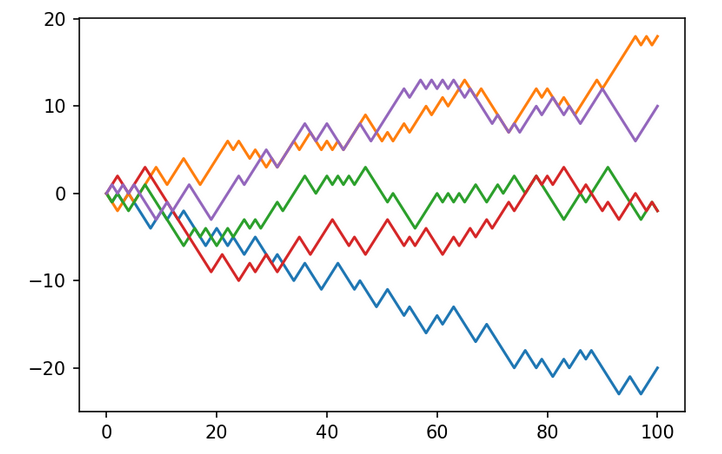Five instantiations of a simple random walk.

It becomes apparent that where you’ll end up after 100 coin flips can differ quite dramatically, even though at every step you face the same 50/50 probability of turning either left or right.

While this process is dominated by chance, it is still interesting to note that as if through magic, the law of large numbers comes into play, and the location of the final states after 100 time steps will follow a distribution that is approximately Gaussian normal with zero mean and variance proportional to the square root of the number of steps. This is easily observed by plotting a histogram of all the final locations of a sample of 500 random walks, and fitting a Gaussian curve to it:

This is only a simple example, and the distributions that we draw from and the spaces that we move through can take on all kinds of shapes and forms, depending on context and application.

While to us random walks might seem conceptually pretty intuitive, it took scientists a long time to figure out that randomness plays an important role if we want to model our understanding world: random walks were only proposed in 1905 by Karl Pearson.

It can be hard for us to wrap around the fact that there is nothing deeper than chance to explain these wildly diverging outcomes, and properties of stochastic processes remain challenging for our minds, which are constantly on the lookout for an explanation (this article goes into our aversion to randomness, and how in turn deliberately injecting noise into our lives could benefit us).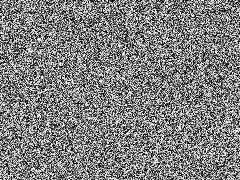White noise. (from Jorge Stolfi, BY-SA 3.0)

After all, Newtonian mechanics were seen as a means to end the reign of randomness, and the backlash against Boltzmann and his proposal of viewing thermodynamics from a statistical lense spoke volumes against our discomfort with allowing too much chance into our theories. But as I mentioned, random walks now find application in many scientific contexts, where, while we assume that at their heart the laws of physics are deterministic (leaving aside quantum effects), a stochastic/statistical description of the system is often more helpful than a deterministic one, e.g. when it comes to the flight of malaria flies, the movement of molecules in a liquid, or the processes in our brain. This conceptual shift has been crucially important, and it remains important to this day: Daniel Kahnemann’s new book Noise gives many great and disconcerting examples of the fact that we are still very bad at acknowledging the important role noisy, random processes play in many aspects of our daily life and how faulty judgments cost us billions of dollars and end up, in the case of the court system, destroying lives.

Now that we have a basic understanding of what a random walk is, we can turn back to Russian poetry.

Assume we didn’t know anything about language or poetry. Then we could take the most abstract approach possible to the lines from Eugene Onegin, and acknowledge that the poetry constitutes a chain of linked “events”, where every letter constitutes an event. One way of discovering structure within them would be by counting “transition probabilities” between events. A very simple kind of event that can take place within a text is the switching between consonants and vowels.

This gives us a list of 4 possible events:

consonant → consonant
consonant → vowel
vowel → vowel
vowel → consonant

Markov, the father of Markov chains, posed the question of how these probabilities look like for a work of text like Eugene Onegin. He removed all interpunctuation and sifted through the first 20000 letters of this famous Russian work of poetry. Fortunately, we don’t need to do this by hand anymore, and can write a simple program that analyzes the quote from above:

`##define lists of consonants and vowelsconsonants=['b','c','d','f','g','h','i','j','k','l','m','n','p','q','r','s','t','v','w','x','y','z']vowels=['a','e','i','o','u']###text with all interpunctuation removedtext="mydreamsmydreamswhathasbecomeoftheirsweetnesswhatindeedhasbecomeofmyyouth"## this function counts all 4 types of transitions and normalizes them by the length of the text:def simple_markov_chain(text):    cv=0    cc=0    vv=0    vc=0        for i in range(len(text)-1):        if text[i] in consonants and text[i+1] in consonants:            cc+=1        if text[i] in consonants and text[i+1] in vowels:            cv+=1        if text[i] in vowels and text[i+1] in vowels:            vv+=1        if text[i] in vowels and text[i+1] in consonants:            vc+=1        return cc/(len(text)-1), vv/(len(text)-1), cv/(len(text)-1), vc/(len(text)-1)`

“My dreams, my dreams! What has become of their sweetness? What indeed has become of my youth?”

This short line of text gives us the following transition probabilities:

consonant → consonant: 0.43
consonant → vowel: 0.11
vowel → vowel: 0.25
vowel → consonant: 0.26

We can now re-write these transitions as a simple diagram, which constitutes a Markov chain: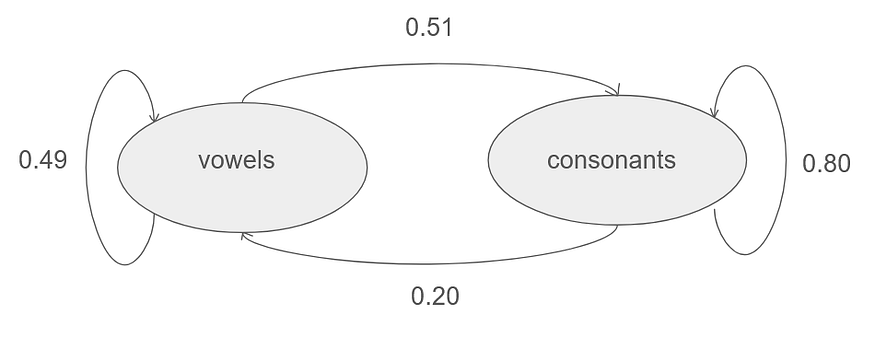The transition probabilities, expressed as a Markov chain. The total probability flow out of each node in the diagram is normalized to one.

This is based only on a small sample from the poem, but Markov observed that these transition probabilities can actually differ between different texts by different authors, and so encode some information about the hidden probabilistic structure of the text.

It is also interesting to note that we can set up these probabilities for different languages, and compare what kind of structures are conserved or are different, questions that are of great interest to (computational) linguists.

One of the benefits of expressing text as a Markov chain is that it gets rid of certain unrealistic independence assumptions that you would get e.g. by drawing letters completely independently: then the probability for a consonant to occur is at roughly 68% (estimated from this sample), but if the current state is a vowel, the probability is only at 50 percent, while for a consonant, it is at 80%.

Thus, the simple Markov chain from above assimilates properties of real text in a more realistic way.

Taking a step back from writing, similar dependency assumptions also make sense in other contexts, as for example for weather: it frequently rains several days in a row, so once you observe rain, another day of rain is more likely than a rain of day following a dry day.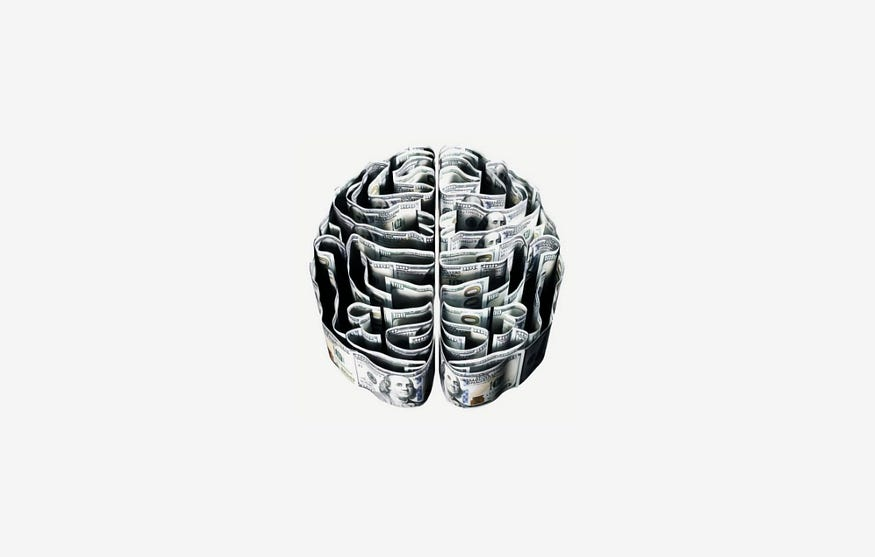Photo by Morgan Housel on Unsplash

The realization that many processes in the world hide a structure that can be expressed in a probabilistic language is quite profound. Modern neuroscience theories like the Bayesian brain hypothesis put this probabilistic, predictive framework at the heart of the brain’s ability to map its understanding of the world. Our brains constantly have to act in environments with partial information, so they don’t have the luxury of modeling everything that is happening, but have to assume that significant parts of the environment are dominated by noise, or at least factors that we can’t predict. Therefore, planning itself becomes a probabilistic process. Just like in the example of the random walk, we all never quite know where we are going to end up. Along every step of the way, there is just too much we don’t know, so we have to factor in uncertainty when making decisions.

Another very useful thing about probabilistic models is that they can be used to construct generative models. As the name already tells, generative models can generate entirely new data after having learned a model, and can therefore also be used to make predictions about future states of a system.

Thus they find their way into all kinds of applications, such as image generation, text generation, audio generation, and much more (I discussed this in my article on How To Make Computers Dream).

For our toy example of a Markov chain, we can implement a simple generative model that predicts a potential text by sampling an initial state (vowel or consonant) with the baseline probabilities (32% and 68%), and then generating a chain of consecutive states, just like we would sample from the random walk introduced earlier:

`import randomconsonants=['b','c','d','f','g','h','i','j','k','l','m','n','p','q','r','s','t','v','w','x','y','z']vowels=['a','e','i','o','u']def markov(iterations):     text=[]    s0=np.random.binomial(1, 0.32, size=None)    if s0 == 1:                    l0=random.choice(vowels)        text.append(l0)    else:        l0=random.choice(consonants)        text.append(l0)                for i in range(iterations):        if s0 == 1:            l=random.choice(vowels)            text.append(l)            s0=np.random.binomial(1, 0.49, size=None)        else:            l=random.choice(consonants)            text.append(l)            s0=np.random.binomial(1, 0.2,size=None)      string=''.join(text)    return string`

Sampling 100 letters gives us the lines:

iewnjgfqxqqlyoqbuoquwpgaavonozbylhjdnjyvtqaakxgmqpiigjauzwvxznqhqkhryiiaxcfblywigbjpshcnnhviqkfrpwai.
– A simple Markov chain

Not quite Russian poetry.

While in principle we now have the power to generate random text, it should be clear that while a very simple Markov chain can mimic some important dependence assumptions within text, the diagram above is way too simplistic to actually lead to meaningful text. Sampling all letters with the same probability, for instance, is highly unrealistic, and won’t lead to many well-formed words.

Another limiting assumption is the so-called Markov property.

This tells us that in order to predict the future state of the system, we only need to know the current state of the system. The past or the future of the system is irrelevant. What counts are only the transitions between states.

What constitutes a state, however, is up for discussion. No one forces us to analyze language on the level of single vowels and consonants. While double consonants are quite frequent, three vowels in a row are extremely rare, so a vowel after two vowels should be much less probable.

We could thus extend our diagram to larger sequences of letters (e.g. vowel vowel → vowel vowel, vowel vowel → consonant vowel …) and thus add more possible states to the Markov chain. This, however, comes at the prize of exponentially increasing the number of states on the chain.

As in many computer science applications, this leads to a trade-off between model size, computational burden, and model quality. To get more towards a realistic version of language that serves a purpose in practice, we can for instance connect every possible word to every other possible word and assign transition probabilities between them, or look at transition probabilities between different letters.

I have a friend who is a good friend and I have been working with you for a few years now. So I hope you are well and I hope you are well.
– My phone

Autocorrect is a familiar companion to most of us. It is also a good example of how predicting transition probabilities and most probable words based on an input can be incredibly useful in everyday life, while we don’t put a lot of burden on sensible long-term predictions (hence the ridiculous nonsensical but grammatically correct loopy sentences once you let autocorrect run rampant).

When it comes to applications of Markov chains, the sky is the limit. Google’s famous PageRank algorithm relies on Markov chains, linking all 40 billion or so pages of the internet among each other, assigning probabilities to each transition.

And if we desire to realistically model language, we need to be mindful of including sufficient long-term dependencies that are, after all, the bread and butter of sensible, human-like texts (state-of-the-art models like GPT-3 work with transformers to express these dependencies more economically). However, there are also more realistic versions of Markov chains for generating language. Markovify, for instance, is a Python package that allows you to read in arbitrary text files and generate new text (this article explains this for Shakespeare tragedies).

Markov chains are useful tools that find applications in many places in AI and engineering. But moreover, I think they are also useful as a conceptual framework that helps us understand the probabilistic structure behind much of reality in a simple and intuitive way, and that gives us a feeling for how scaling up this probabilistic structure can lead to powerful models of the world.